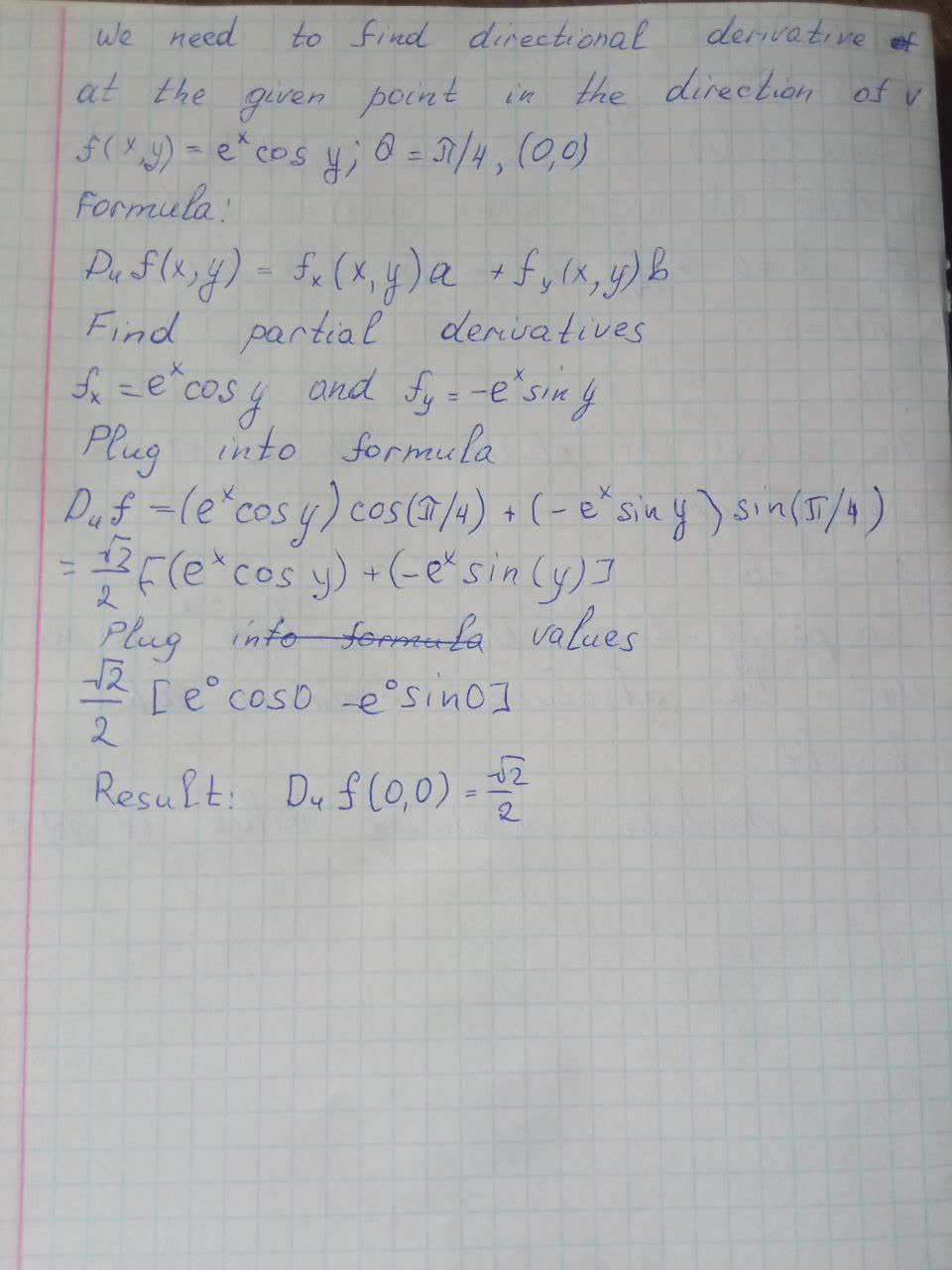# Find the directional derivative of f at the given point in the direction indicated by the angle \theta. f(x,y)=e^x\cos y,(0,0),\theta=\frac{\pi}{4}Ernstfalld 2021-09-08 Answered
Find the directional derivative of f at the given point in the direction indicated by the angle $\theta .f\left(x,y\right)={e}^{x}\mathrm{cos}y,\left(0,0\right),\theta =\frac{\pi }{4}$
You can still ask an expert for help

• Questions are typically answered in as fast as 30 minutes

Solve your problem for the price of one coffee

• Math expert for every subject
• Pay only if we can solve itDaphne Broadhurst
At the given condition: